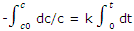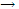Describe First Order Rate Equation

The integrated forms of the first order rate equations are conveniently used to compare concentration time results with this rate equation.

Rate equations show the dependence of the rate of the reaction on concentration can be integrated to give expressions for the dependence of the concentrations on time. We generally use the integrated rate equation that is obtained to deduce the order of a reaction.

A first order reaction is one for which, at a given temperature, the rate of the reaction depends only on the first power of the concentration of a single reacting species. If the concentrations of this species is represented by c (for solutions, the units of moles per litre are ordinarily used), and if the volume of the system remains essentially constant during the course of the reaction, the first order rate equation can be written

-dc/dt = kc

The rate of constant k is then a positive quantity and has the units of the reciprocal of time.

Integrated rate equation: the experimental results obtained in a study of the rate of a reaction are usually values of c or some related to c at various times. Such data can best be compared with the integrated form of the first order rate equation. If the concentration at time t = 0 is c0, and if at some later time t the concentration has fallen to c, the integration givesWith -In (c/c0) = In (c0/c), the integration can be written as

In c0/c = kt

Sometimes a more convenient form is

In c = -kt + In c0

A reaction can therefore be said to be first order if a plot of In (c0/c) or In c versus t gives a straight line. If a straight line is obtained, the slope of the line can be used to give the value of the rate constant k. an alternative to this graphical procedure is the calculation of a value of kfrom the individual measurements of c at the various times t, for example. The reaction is classified as first order if all the data lead to essentially the same values for k, that is, if it is satisfies with k as a constant.

Example: the rate of conversion of tert-butyl bromide to tert-butyl alcohol, (CH3)3CBr + H2O(CH3)3COH + HBr, has been studied and some concentration-time results are found in table given below. Verify that the reaction is first order, and deduce the values of the rate constant at the two temperatures.

Solution: from the data at each temperature we calculate In (c0/c) values. Then the graphical display shows a plot of In (c0/c) versus t is constructed. The straight lines, each going through the origin show that at both temperatures the data conform to the integrated first order relation. The slopes give the values of the rate constants

K = 0.00082 min-1 = 0.137 × 10-4 s-1 [25°C]

K = 0.0142 min-1 = 2.37 × 10-4 s-1 [50°C]

Concentration of tert butyl bromide as a function of time for the reaction (CH3)3CBr + H2O(CH3)3COH + HBr in a 10% water, 90% acetone solvent

 At 25°C At 50°C
 Time, h (CH3)3CBr, Mol L-1 Time, min (CH3)3CBr, Mol L-1 0 0.1039 0 0.1056 3.15 0.0896 9 0.0961 6.20 0.0776 18 0.0856 10.0 0.0639 27 0.0767 13.5 0.0529 40 0.0645 18.3 0.0353 54 0.0536 26.0 0.0270 72 0.0432 30.8 0.0207 105 0.0270 37.3 0.0142 135 0.0174 43.8 0.0101 180 0.0089

#### Related Questions in Chemistry

• ##### Q :Describe characteristics of halides and

Halides characteristics

• ##### Q :Mass percent Help me to go through this

Help me to go through this problem. 10 grams of a solute is dissolved in 90 grams of a solvent. Its mass percent in solution is : (a) 0.01 (b) 11.1 (c)10 (d) 9

• ##### Q :Product of HCl Zn Illustrate  the

Illustrate  the product of HCl Zn?

• ##### Q :Vapour pressure related question Help

Help me to solve this question. Which of the following is incorrect: (a) Relative lowering of vapour pressure is independent (b)The vapour pressure is a colligative property (c)Vapour pressure of a solution is lower than the vapour pressure of the solvent (d)The

• ##### Q :Problem based on molality of glucose

Select the right answer of the question. If 18 gm of glucose (C6H12O6) is present in 1000 gm of an aqueous solution of glucose, it is said to be: (a)1 molal (b)1.1 molal (c)0.5 molal (d)0.1 molal

• ##### Q :Einsteins mass energy relation In

In Einstein’s mass energy relation e = mc2 for what is c employed or why is light needed for the reactions. As the reactions are with the help of neutrons?

• ##### Q :Theory of three dimensional motion

Partition function; that the translational energy of 1 mol of molecules is 3/2 RT will come as no surprise. But the calculation of this result further illustrates the use of quantized states and the partition function to obtain macroscopic properties. The partition fu